# Get these flashcards, study & pass exams. For free! Even on iPhone/Android!

## AQA Module 3 Foundation (9 Cards)

What is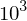1000or '10 cubed' means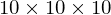What are square numbers?
Square numbers are the result of squaring of a number.

Example:

9 is a square number because 3 x 3 = 9
What is 14 out of 20 as a percentage?
70%

Calculator method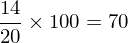Non-calculator method

Make it an equivalent fraction with a denominator of 100 (in this case by multiplying the top and bottom by five).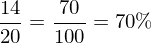How do you multiply fractions together?
Just multiply the numerators together and multiply the denominators together.

Example: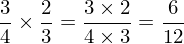And cancel it down if you can - so this would be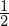How do you divide fractions?
Flip the second fraction and then multiply the 2 fractions together (times the numerators together and times the denominators together).

Example: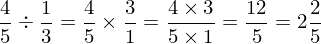*Here we've given our answer as a mixed number too.
What is a factor?
A number that you can divide another number by.

Example:
1, 2, 3, 4, 6 and 12 are all factors of 12 because you can divide 12 by all these numbers.
What is a multiple?
A multiple of a number is any number in that times table.

Example:
Multiples of 10 are 10, 20, 30, 40, 50, ..., ..., ...

Multiples of 4 are 4, 8, 12, 16, 20, 24, ..., ..., ...
How can I find 37% of 420 with a calculator
Method 1 - Using the percentage button
Simply enter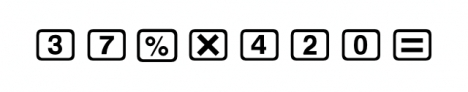Method 2 - Change to a decimal first
37% is 0.37 as a decimal so enter...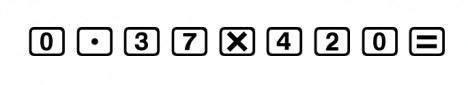Either way you should get 155.4
Estimate the answer to 8.78 x 3.92 - 5.24
31

Round each number to one significant figure...

9 x 4 - 5 = 36 - 5 = 31

Remember
When the questions says estimate you will not get marks if you just work it out so do what it says - round the numbers first!!!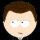Flashcard set info:
Author: mrfoxton
Main topic: Mathematics
Topic: General
Published: 07.02.2010

Card tags:
All cards (9)
no tagsReport abuse